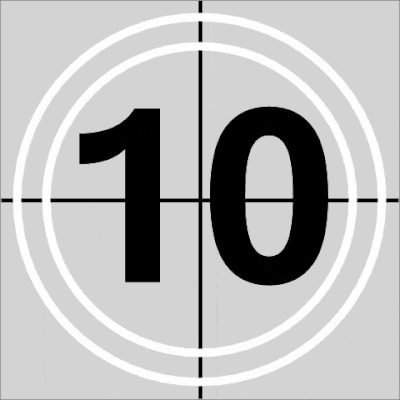## Fun With SAS ODS Graphics: "Happy New Year!" Film Leader CountdownHappy New Year! (note: GIF compressed with compressor.io)

To mark the new decade, here's a quick-and-dirty SAS ODS Graphics take on a film leader countdown. Happy New Year, all!

``````* Fun w/SAS ODS Graphics: "Happy New Year!" Film Countdown Leader (see en.wikipedia.org/wiki/Film_leader);

data points(keep=counter angle text x y tx ty vx vy);      * Counter=10, 9, ..., 1;
pi=constant('pi');                                         * Angle=degrees of slices (0 to 360 by 15);
retain tx ty 0;                                            * x/y=Polygon coordinates, tx/ty=text, vx/vy=vector;
do counter=10 to 1 by -1;                                  * Countdown seconds, 10 to 1;
text=put(counter,2.);                                    * Format econds remaining;
do angle=0 to 360 by 15;                                 * Plot every 15 degrees beginning at top;
x=0; y=0; output;                                      * Start drawing shaded polygon at center (0,0);
do i=0 to angle by 1;                                  * Add more points to shaded plygon (one point/degree);
output;
end;
end;
end;
run;

options nobyline;
proc template;                                             * Film countdown leader chart definition;
define statgraph filmtemplate;                             * Composite of scatter, polygon, vector, ellipse, text plots;
layout overlayequated /
walldisplay=none backgroundcolor=lightgrey opaque=true
commonaxisopts=(tickvaluelist=(-1 1) viewmin=-1 viewmax=1) /* Cutoff window at -1 and 1 to trim" polygon area */
xaxisopts=(display=none offsetmin=0.000 offsetmax=0.000 viewmin=-1 viewmax=1)  /* Suppress axes/ticks/values */
yaxisopts=(display=none offsetmin=0.000 offsetmax=0.000 viewmin=-1 viewmax=1);
scatterplot x=x y=y / markerattrs=(size=0); * Ensure at least some data is plotted;
polygonplot x=x y=y id=angle / fillattrs=(color=gray) display=(fill) label=none; * Shaded "radar sweep" area;
vectorplot x=vx y=vy xorigin=0 yorigin=0 / arrowheads=false lineattrs=(color=black thickness=4pt); * "Radar sweep" hand;
referenceline x=0 / lineattrs=(color=black thickness=4pt); * Line bisecting x axis;
referenceline y=0 / lineattrs=(color=black thickness=4pt); * Line bisecting y axis;
ellipseparm semimajor=.92 semiminor=.92 xorigin=0 yorigin=0 slope=0 / outlineattrs=(color=white thickness=8pt); * Outer circle;
ellipseparm semimajor=.77 semiminor=.77 xorigin=0 yorigin=0 slope=0 / outlineattrs=(color=white thickness=8pt); * Inner circle;
textplot x=tx y=ty text=text / textattrs=(size=216pt color=black weight=bold) strip=true contributeoffsets=none; * Seconds remaining;
endlayout;
endgraph;
end;
run;
* Create animated GIF film countdown leader followed by Happy New Year! message;
ods _all_ close;                                           * Animated GIF setup;
options papersize=('5 in', '5 in') printerpath=gif animation=start
nodate nonumber animloop=YES NOANIMOVERLAY;
ods graphics / width=5in height=5in imagefmt=GIF;

options animduration=.04;                                   * 25 frames per second;
proc sgrender data=points template=filmtemplate;            * Generate countdown frames using BY statement;
by descending counter angle;
run;

data year;                                                  * Text for Happy New Year message;
retain tx ty 0 msg "2020" tx2 0 ty2 .9 msg2 "HAPPY NEW YEAR!";

options animduration=2;                                     * Generate Happy New Year! message frame (2 seconds);
proc sgplot data=year aspect=1 noborder opaque nowall noautolegend;
styleattrs backcolor=black;
text x=tx y=ty text=msg / textattrs=(size=128pt color=white weight=bold) contributeoffsets=none;
text x=tx2 y=ty2 text=msg2 / textattrs=(size=30pt color=white weight=bold) contributeoffsets=none position=bottom;
xaxis display=none values=(-1 0 1); yaxis display=none values=(-1 0 1);
run;

options printerpath=gif animation=stop;                     * Animated GIF wrapup;
ods printer close;``````DanH_sas